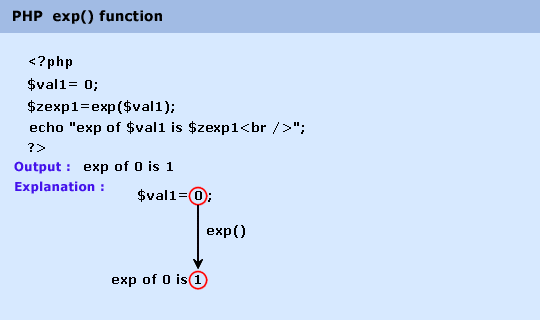# Education is not limited to just classrooms. It can be gained anytime, anywhere... - Ravi Ranjan (M.Tech-NIT)

## PHP : exp() function

Description

The exp() function is used to calculate the  exponent of e. 'e' is the base of the natural system of logarithms (approximately 2.718282).

Version

(PHP 4 and above)

Syntax

exp(x)

Parameter

Name

Description

Required /
Optional

Type

x

Specifies the exponent.

Required

Float

Return value

'e' raised to the power of x.

Value Type : Float.

Pictorial PresentationExample :

1. \$val1= 0;

2. \$val2=1;

3. \$val3=2.5;

4. \$val4=10;

5. \$zexp1=exp(\$val1);

6. \$zexp2=exp(\$val2);

7. \$zexp3=exp(\$val3);

8. \$zexp4=exp(\$val4);

9. echo "exp of \$val1 is \$zexp1
";

10. echo "exp of \$val2 is \$zexp2
";

11. echo "exp of \$val3 is \$zexp3
";

12. echo "exp of \$val4 is \$zexp4
";

13. ?>

Output :

exp of 0 is 1 exp of 1 is 2.71828182846 exp of 2.5 is 12.1824939607 exp of 10 is 22026.4657948